• No products in the cart.

# MAGNETISM A-LEVEL PHYSICS

##### This Unit is about Magnetic Fields and how best they work.

MAGNETISM

The magnetic field:

A magnetic Field is a region or space in which:

1. a magnetic dipole (magnet) experiences a force.
2. a current carrying conductor experiences a force or a moving charge experiences a force
3. an emf is induced in a moving conductor

Field lines are used to represent the direction and magnitude of the magnetic field. The strength of the magnetic field is proportional to the density of the field lines.

The direction o the magnetic field is represented by the magnetic field lines. The magnetic field lines are taken to pass through the magnet, emerging from the North Pole and returning via the South Pole. The lines are continuous and do not cross each other.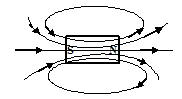Magnetic fields due to a straight wire carrying current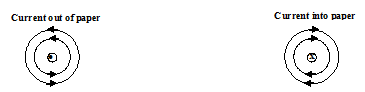Maxwell’s right hand rule:

This is used to find the direction of the field.

If one grasps the current carrying straight wire in the right hand with the thumb pointing in the direction o current, then the fingers curl pointing in the direction of the magnetic field.

Magnetic field due to a current carrying circular coil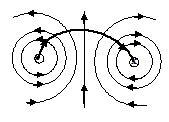Magnetic fields near the center of the circular coil are uniform hence the magnetic field lines are nearly straight and parallel.

Magnetic fields due to a solenoid

A solenoid can be viewed as consisting o many circular coils, wound very closely to each other.Use right hand grip to determine direction of field. (fingers show direction of current; thumb direction)

In the middle of the solenoid, the magnetic field lines are parallel to the axis of the solenoid. At the ends of the solenoid, the lines diverge from the axis. The magnetic field due to a current carrying solenoid resembles that of a bar magnet. The polarities of the field are identified by looking at the ends of the solenoid. If current flow is clockwise, the end of the solenoid is South pole, and if anticlockwise then it is North pole.

Magnetic force due to a current carrying conductor in the magnetic field

When a current carrying conductor is placed in the magnetic field, a force is exerted on it. Experiments show that the magnitude o the force exerted is proportional to:-

1. the current I in the conductor
2. the length, l, of the conductor
3. the strength of the magnetic field by a quantity called magnetic flux density, B.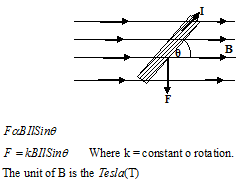The Tesla is the magnetic flux density in a magnetic field when the conductor of length 1m, carrying a current of 1A, at right angles to the magnetic field is 1N.

When B =1T then F = 1N, I = 1A, = 1m, = 900

1 = k x1 x 1 x 1 x 1

k = 1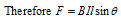The direction of the force carrying current in the magnetic field is determined using Fleming’s left hand rule.

If the thumb, the 1st finger and the 2nd finger of the left hand are positioned mutually at right angles, with the 1st finger pointing in the direction of the magnetic field and the 2nd finger pointing in the direction of current, then the thumb points in the direction o the force.

Force of a charge in a magnetic field.

Consider a wire o length l, carrying a current I, in a uniform magnetic field o flux density B.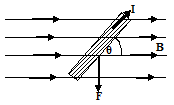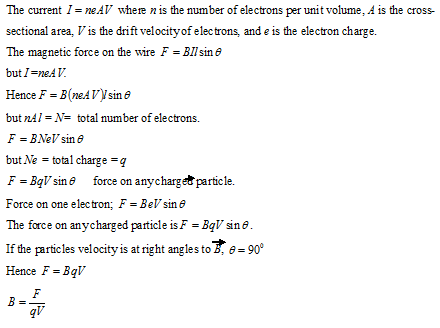Hence magnetic flux density B at a point in a magnetic field, is the force exerted on a charge o 1C, moving with a velocity of 1ms-1, at right angles to the magnetic field.

Motion of a charged particle in a uniform magnetic field

Suppose a positively charged particle is projected with velocity V at right angles to a uniform magnetic field o flux density B.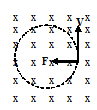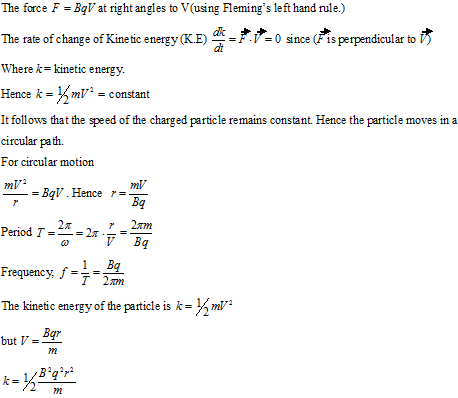Torque on a current carrying coil in a uniform magnetic field

Consider a rectangular coil carrying a current I, in a uniform magnetic field of flux density B. Suppose the plane o the coil makes an angle with the magnetic field.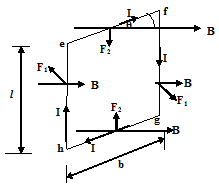By Fleming’s left hand rule, the force on the side ef on the coil is , vertically downwards, whereas the force on hg is , vertically upwards.

The forces F2, compress the coil and are resisted by the coil’s rigidity. On the side eh, into the plane. The force on fg is perpendicularly out of the plane o the figure. The forces on eh and fg are equal and opposite and hence constitute a couple. The moment of this couple, is the torque.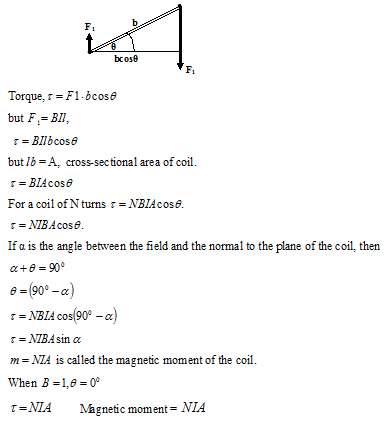Therefore m, the magnetic moment is the torque exerted on a coil when it is placed with its plane parallel to a field of magnetic flux density 1T.

THIS VIDEO EXPLAINS MORE ABOUT MAGNETIC FIELDS

Yaaka DN © Ultimate Multimedia Consult